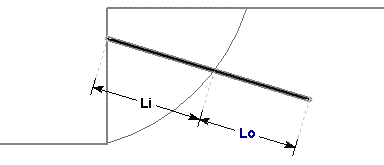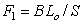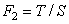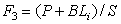Join one of our upcoming courses taking place around the world! Explore now

# Soil Nail

The Soil Nail support type can be used to model soil nail slope reinforcement. Note:

• A Soil Nail is assumed to be fully bonded along its entire length
• The Soil Nail support type in Slide2 is equivalent to Grouted Tieback support with Bond Length = 100%
• The Soil Nail support type in Slide2 is differentiated from the Grouted Tieback for user convenience. However, the user should be aware that, as far as the Slide2 implementation is concerned, a Grouted Tieback with Bond Length = 100% would behave exactly the same as a Soil Nail, all other parameters being equal.
• A Soil Nail is NOT equivalent to the Grouted Tieback with Friction support type in Slide2.
• Soil Nails can also be used to model pile or micropile support by using the optional Shear or Compression options as described below.
Make sure that you have read the Overview of Support Implementation topic, for important information which applies to all support models in Slide2.

## Tensile Capacity

The Tensile Capacity entered for a Soil Nail, represents the maximum tensile capacity of an individual soil nail. This is the capacity of the soil nail itself (e.g. steel tensile capacity), independent of the plate capacity or the bond capacity. Units are Force.

## Plate Capacity

The Plate Capacity is the maximum load which can be sustained by the plate assembly which connects the soil nail to the slope. Units are Force.

## Shear Capacity

The Shear Capacity is optional and allows you to account for shear failure through the bolt cross-section (i.e. the force required to shear the bolt perpendicular to its axis). Units are Force. See below for implementation details.

## Compression Capacity

The Compression Capacity is optional and allows you to account for the failure of the bolt in compression. This can be useful for modelling compression piles, for example. Units are Force. See below for implementation details.

## Out of Plane Spacing

The spacing between soil nails in the out-of-plane direction (i.e. along the slope), measured from center to center.

## Force Application

See the Force Application topic for a discussion of the significance of Active and Passive support force application in Slide2.

## Force Orientation

When the support begins to take on a load, due to displacements within the slope, the direction of the applied support force can be assumed to be:

• Tangent to Slip Surface
• Bisector of Parallel and Tangent (i.e. at an angle which bisects the tangent to slip surface orientation, and the parallel to reinforcement orientation)
• Parallel to Reinforcement
• Horizontal
• User-Defined Angle (i.e. the user may specify an angle, measured from the positive horizontal direction)

If you are using the Shear Capacity option, the force may be applied at some angle to the bolt axis, corresponding to the resultant of the shear and tensile components (see below for details).

## Pullout Strength

For a Soil Nail, the Pullout Strength is expressed as a Force per unit Length.

The length units in this case, refer to the length along the soil nail. The Bond Strength determines the pullout and/or stripping force which can be generated by a soil nail.

## Material Dependent Pullout Strength

See the Grouted Tieback topic for details, as the procedure is the same.

## Implementation of Soil Nail Support in Slide2

Consider a soil nail which intersects a slip surface, as shown below.Li = length of soil nail within sliding mass

Lo = length of soil nail embedded beyond slip surface

Soil Nail Parameters

B = Bond Strength (force / unit length of soil nail)

S = Out of Plane Spacing

T = Tensile Capacity (force)

P = Plate Capacity (force)

At any point along the length of the soil nail, there are 3 possible failure modes which are considered:

1. Pullout (force required to pull the length Lo of the nail out of the slope)
2. Tensile Failure (maximum axial capacity of the soil nail)
3. Stripping (slope failure occurs, but nail remains embedded in slope)

The maximum force which can be mobilized by each failure mode, PER UNIT WIDTH OF SLOPE, is given by the following equations:

Pullout:Eqn.1

Tensile:Eqn.2

Stripping:Eqn.3

At any point along the length of a soil nail, the force which is applied to the slip surface by the soil nail, is given by the MINIMUM of these three forces.

Applied Force = min (F1, F2, F3) Eqn.4

• In order for stripping to occur, the Plate Capacity must be exceeded. The Plate Capacity is included in the stripping force equation, and added to the shear capacity along the length Li.
• If the Soil Nail Pullout Strength is specified as Material Dependent, then the Pullout Force and Stripping force, are determined by integrating along the lengths Lo and Li, to determine the force contributed by each segment of the soil nail which passes through different materials.

A typical Soil Nail Force diagram, which exhibits all three failure modes, is shown below. In this case, the Plate Capacity is less than the Tensile Capacity, and therefore "stripping" is a possible failure mode. If the Plate Capacity is greater than or equal to the Tensile Capacity, then stripping cannot occur, and the Soil Nail Force diagram will be determined only by the Tensile and Pullout failure modes.

### Implementation of Shear Capacity

If the Shear Capacity option is selected, an additional force vector perpendicular to the bolt direction, and opposite to the direction of failure, is added to the overall bolt capacity vector, determined by the methods described above. You’ll see this in the Interpreter when you show the slice data. The support force at the base of the slice is no longer parallel to the support but angled in a direction opposite to the slip direction. The Shear Capacity option may be useful if you expect that the support forces are not purely tensile, but may include a transverse shear component as well. This depends on the orientation of the support relative to the slip surface direction.